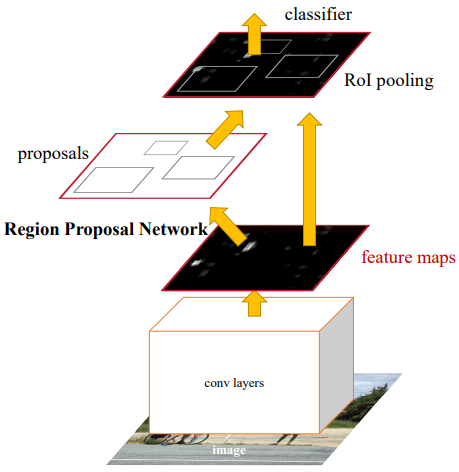# Day 80(DL) — Object detection (Fast R-CNN Vs Faster R-CNN)

Fast R-CNN: Fast R-CNN is an enhanced variant of R-CNN, designed for object detection. It implements VGG16 as the backbone network, trains it 9 times faster than R-CNN, while 213 times faster at test-time. Usually, the object detection task is more challenging compared to the image classification. The two prime reasons being (1) ’n’ number of region proposals must be processed (2) These ROIs(region of interest) depict only a rough estimate of the localization, which must be distilled in order to get the final BB boxes.

• The main differentiating factor between the R-CNN & Fast R-CNN is the number of times CNN is called. In the case of R-CNN, each region proposal has to be fed as input to the CNN(2000 times). Whereas, Fast R-CNN takes an entire image as an input for CNN processing(one single process). After that, the network produces feature maps(via convolutional and max-pooling layers), ROIs are built(extracted) from the feature maps. This is usually done by projecting the ROIs from the selective search algorithm on the feature map by considering the downsampling process that happened in CNN.
• Since we need to have a constant size output from CNN before giving it to the fully connected layer, the RoI(max) pooling layer is employed for the warping. Irrespective of the input size to the pooling layer, the output takes a fixed shape.
• Even though, fast R-CNN outperforms R-CNN still it has the drawback of using an algorithm to select the ROIs(time-consuming). The next step towards better speed resulted in Faster R-CNN.

Faster R-CNN: The principle behind the algorithm is to make the region proposal process learnable by introducing a network called RPN(regional proposal network). RPN is another CNN for predicting the object bounds and objectness scores at each position. The RPN merged with Fast R-CNN is utilized for object detection.Fig 2 — shows faster R-CNN stages taken from original paper
• The idea here is to share the convolutional feature maps, designed for object detection, with the region proposal process. The feature maps from the initial CNN are fed into the Region proposal network(another CNN) that comprises some more convolutional layers for predicting the region proposals. RPNs are capable of proposing ROIs covering a wide range of scales and aspect ratios.
• Since the feature maps are shared between the RPN and the object detection, the training process happens in alternate steps of optimizing both(RPN & the object detection) while maintaining the proposed regions.

Region Proposal Networks(RPN): The network accepts input of any dimension to produce multiple object proposals(in a rectangular shape) associated with an objectness score. Input to the RPN is from another CNN architecture(such as VGG-16/ZFNet or any pre-trained architectures) which basically are feature maps of the input image. In order to create region proposals, a small sliding window of size n x n (with n =3 ) will be slid over the input feature maps. This is followed by two identical 1 x 1 convolution layer for predicting the objectiveness and the region proposals.

Say suppose if we have k boxes, then the output of the class layer(objectiveness) will be 2k scores. Since we need to output two probabilities indicating whether it contains an object or not(objectiveness). The other output is the region proposals in the form of coordinates which has the count of 4k coordinates. As every box in the ‘k’ suggestion corresponds to a proposed region indicated by 4 coordinate values.

Anchor boxes: Now we need to gain knowledge on anchor boxes to know more about the region proposals. When we slide the window every time on the feature map, ‘k’ set of anchor boxes are created w.r.t the central point in the sliding window frame. The number of anchor boxes is defined based on the varying scales and the aspect ratios. Let’s consider ‘w’ as width of the feature map and ‘h’ as the height. Then, we’ll have w * h * k anchor boxes. In the paper, the number of anchor boxes chosen is 9 based on different aspect ratios such as 1:1, 1:2, 2:1 and shapes (128, 256 and 512).

The idea here is, instead of making RPN learn the bounding boxes completely from scratch, initial bounding boxes are created around the point with the shapes and aspect ratio as mentioned above. Now, the network only has to learn how much value of the coordinates has to be adjusted to reach the ground truth. This technique speed up the learning process.

The anchor boxes with less objectiveness score will be eliminated when the RPN gets trained during backpropagation. The finalised boxes will be the regional proposals that get superimposed on the original feature map from the initial backbone network. After passing into the ROI pooling layer, the bounding boxes and the classification outputs will be predicted.

This is only a very high-level overview of faster R-CNN. We’ll go in-depth with the actual network formation.

https://tryolabs.com/blog/2018/01/18/faster-r-cnn-down-the-rabbit-hole-of-modern-object-detection/